Refer to our Texas Go Math Grade 2 Answer Key Pdf to score good marks in the exams. Test yourself by practicing the problems from Texas Go Math Grade 2 Module 18 Assessment Answer Key.

Concepts and Skills

Look at the clock hands. Write the time.

Question 1.Answer: The time on the clock is 6:40

Explanation: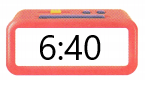The hour hand is between 6 and 7
and the minute hand is on 8 , which makes 40 minutes on the clock ,
for example , if the minute hand is on 6 , makes 30 minutes
but here it is on 8 that means add 10 minutes to the 30 minutes which results in 40 minutes
Making it as 6:40 on the clock

Question 2.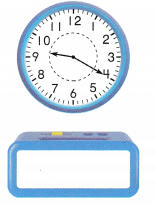Answer: The time on the clock is 9:20

Explanation:The hour hand is between 9 and 10
and the minute hand is on 4 , which makes 20 minutes on the clock ,
Making it as 9:20 on the clock

Question 3.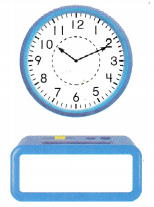Answer: The time on the clock is 10″:10

Explanation: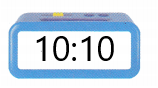The hour hand points at 10
and the minute hand is on 2 , which makes 10 minutes on the clock ,
Making it as 10:10 on the clock

Look at the clock hands. Write the time. TEKS 2.9.G

Question 4.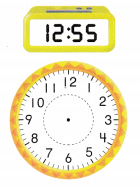Answer:Explanation:
The hour hand is between 12 and  close to 1
and the minute hand is on 11 , which makes 55 minutes on the clock ,
Here 6o minutes makes an hour, so 55 minutes means 5 minutes less than a hour
Making it as12:55 on the clock

Question 5.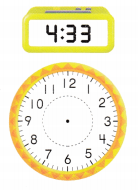Answer: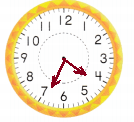Explanation:
The hour hand points between 4 and 5
The minute hand points between 6 and close to 7 That is 2 minutes less than 35 minutes.
Making it to 4:33

Question 6.Answer: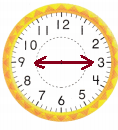Explanation:
The hour hand points at 9
and the minute hand is on 3, which makes 15 minutes on the clock
That is adding up the 5 minutes three times can make a whole 15 minutes.
Making it as 9:15 on the clock

Write the time. Then circle a.m. or p.m. TEKS 2.9.G.

Question 7.
go to science classAnswer: Go to science class at 10:30 a.m.

Explanation:before noon , that is before 12 we call it as a.m. and after 12 we call it as p.m.
The hour hand is between 10 and 11
and the minute hand is on 6, which makes 30 minutes on the clock
Making it as 10:30 on the clock

Question 8.
look at the moon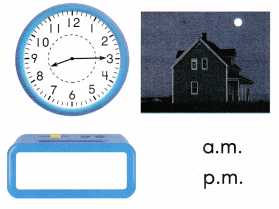Answer: The time is 8:15 p.m.

Explanation:before noon , that is before 12 we call it as a.m. and after 12 we call it as p.m.
The hour hand is pointed at 8
and the minute hand is on 3, which makes 15 minutes on the clock
That is adding up the 5 minutes three times can make a whole 15 minutes.
Making it as 8:15 on the clock

Texas Test prep

Fill in the bubble for the correct answer choice.

Question 9.
The clock shows the time that music class starts. At what time does music class start? TEKS 2.9.G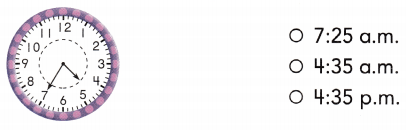Answer: The music class starts at 4:35

Explanation:
Past noon is known as p.m.
The hour hand is between 4 and 5
and the minute hand is on 7 , which makes 35 minutes on the clock ,
for example , if the minute hand is on 6 , makes 30 minutes
but here it is on 7 that means add 5 minutes to the 30 minutes which results in 35 minutes
Making it as 4:35 on the clock

Question 10.
Luke started soccer practice at the time shown on the clock. Which clock shows this same time? TEKS 2.9.G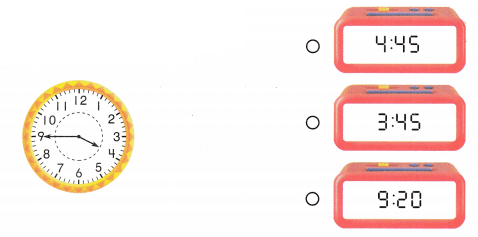Answer: Luke started soccer practice at  3:45

Explanation:
The hour hand is between 3 and 4
and the minute hand is on 9 , which makes 45 minutes on the clock ,
for example , if the minute hand is on 6 , makes 30 minutes
but here it is on 9 that means add 15 minutes to the 30 minutes which results in 45 minutes
Making it as 3:45 on the clock

Question 11.
The train arrives to the station at the time shown on the digital clock. Which clock shows this same time?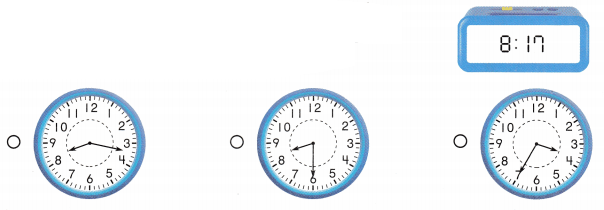Answer:Explanation:
The hour hand points between 8 and 9
The minute hand points between 3 and 4 which means around 20 minutes
That is less than 3 minutes is 17 minutes
Making it 8:17
Thus, The train arrives to the station at 8:17

Question 12.
Art class started at the time shown on the clock. At what time did art class start? TEKS 2.9.G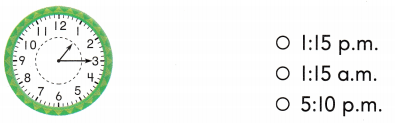Answer:  Art class started at 1:15 p.m.

Explanation:
Past noon is known as p.m.
The hour hand is between 1 and 2
and the minute hand is on 3, which makes 15 minutes on the clock
That is adding up the 5 minutes three times can make a whole 15 minutes.
Making it as 1:15 on the clock.

Scroll to Top